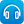Close message Scootle will be undergoing maintenance between 17:00 to 19:00 on 25 September 2023. You may experience intermittent connection during this time. We apologize for any inconvenience caused.

# Mathematics / Year 6 / Number and Algebra / Fractions and decimals

Version 8
Curriculum content descriptions

Multiply decimals by whole numbers and perform divisions by non-zero whole numbers where the results are terminating decimals, with and without digital technologies (ACMNA129)

Elaborations
• interpreting the results of calculations to provide an answer appropriate to the context
General capabilities
• Numeracy Numeracy
• ICT capability Information and Communication Technology (ICT) capability
ScOT terms

Division,  Multiplication,  Decimals

## Refine by topic

Related topic### Sites2See – number for primary

Selected links to a range of interactive online resources for the study of number in Foundation to Year 6 Mathematics.These seven learning activities, which focus on 'open-ended tasks' using a variety of tools (software) and devices (hardware), illustrate the ways in which content, pedagogy and technology can be successfully and effectively integrated in order to promote learning. In the activities, teachers use investigations in order ...### Fraction basics - Easy & Effective Fractions Tutor - iTunes app

Learn about the core concepts of fractions through 12 animated clips. View the clips on the topic that you want to learn about. These clips will help build a string foundation in fractions. Free when reviewed on 12/5/2015.### Circus towers: square stacks

Work out how many acrobats are needed to form square-shaped human towers. Start by building a square tower with four acrobats: two acrobats in the base layer and two acrobats standing on their shoulders. Examine a table and graph of the total number of acrobats in the towers. Predict the number of acrobats needed to build ...### The foul food maker: go figure

This tutorial is suitable for use with a screen reader. It explains how the use of simple words can describe the likelihood of everyday events. How likely is an event: certain, likely, equal chance, unlikely or certainly not? Answer some questions using these words and then build your own examples. Learn how to describe ...### Home energy use

Reducing carbon dioxide emissions and sustainable energy use and are two of the major issues facing the world today. This project explores energy use in homes, and compares individual energy use with the class average and calculate and graph CO2 emissions.This is a video demonstration, with audio commentary, of the procedure of adding decimal fractions. In the commentary, the presenter emphasises the links with the underpinning concepts of place value. Practice questions, limited to addition of decimal fractions involving tenths and hundredths, are presented with answers, ...### Subtracting decimals

This is a video demonstration, with audio commentary, of the procedure for pen-and-paper subtraction of decimal fractions. In the commentary, the presenter emphasises the links with the underpinning concepts of place value. Practice questions, limited to subtraction of decimal fractions involving tenths and hundredths, ...### What's the problem: nature: level 2

Star as a contestant in a quiz show! Select three worded mathematical problems, about nature, from two categories: 'plants' or 'animals'. Identify the correct answer for each step in the problem-solving process. Examine the suggestions made by the panel to help you. Collect a prize based on your score.### Decimals and fractions

This is a video demonstration of converting decimal fractions to simplified fractions. In the commentary, the presenter highlights the relevance of understanding and applying place value concepts, including the efficiency in initially interpreting the fractional part of the decimal fraction as a single fraction. Practice ...### Sites2See: Patterns and Algebra

Selected links to a range of interactive online resources for the study of patterns and algebra in Foundation to Year 6 Mathematics.### Summing Decimals

An interactive resource in which students learn how to add and subtract numbers that have up to two decimal places.### Imperial pie

This activity involves making a cake using a recipe in which the quantities of the ingredients required are measured using a variety of imperial units. To complete the recipe, students need to convert the imperial units to metric units in order to be able to use their metric measuring instruments. The activity serves to ...### The Numberline

An interactive tool that can help students explore a number line, including points representing integers, fractions and decimals### Ordering Decimals

An animated tutorial about ordering numbers with up to 3 decimal places. An interactive quiz is included.### Patterns, primes and Pascal's Triangle

Are you intrigued by patterns? Check out Vi Hart as she explains how to visualise patterns in prime numbers, using Ulam's Spiral. Watch as Vi creates patterns, using Pascal's Triangle to explore relationships in number. See what happens when she circles the odd numbers. What rule does she use to create the final pattern?### Numbers Count: What are factors?

What are factors? Watch as the jelly babies in this clip show you! What are the factors of 12? How many factors does the number 11 have? Try explaining to a friend what a prime number is.### Self Improvement Wednesday: The beauty of prime numbers

A prime number is a number that only has two factors: one and itself. Listen to Adam Spencer and Richard Glover discussing prime numbers. They cover how we define these numbers and how and why prime numbers are widely used in internet encryption.### What is a quarter?

What is a quarter? You get quarters when you divide a whole into four equal parts. Each one of these four parts is a quarter. Watch this great explainer produced by Monique in collaboration with ABC Splash and see how she explains quarters.### Catalyst: Prime numbers and unbreakable codes

Imagine if anyone was able to read all our secret, encrypted messages and information. Watch and find out how scientists at the Australian National University are developing a new encryption system using quantum physics and quantum computing.